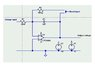# Quantity referred to as 'self-capacitance

bbh2808
Hi all!

I've got a question about the quantity referred to as 'self-capacitance'. From what I can gather, self-capacitance is the amount of charge necessary to generate a 1 Volt E-field between an arbitrary surface and a ground plane @ infinity. This doesn't make sense to me, but that's why I'm hoping someone here can help me understand it!

Typically when people talk about capacitance, it means 'mutual capacitance', the amount of charge @ the surface of the conductor/volt between two conducting surfaces, which makes a lot more sense to me...

How would one measure the 'self-capacitance' of a conductor of arbitrary geometry?

b.shahvir

Hi all!

I've got a question about the quantity referred to as 'self-capacitance'. From what I can gather, self-capacitance is the amount of charge necessary to generate a 1 Volt E-field between an arbitrary surface and a ground plane @ infinity. This doesn't make sense to me, but that's why I'm hoping someone here can help me understand it!

Typically when people talk about capacitance, it means 'mutual capacitance', the amount of charge @ the surface of the conductor/volt between two conducting surfaces, which makes a lot more sense to me...

How would one measure the 'self-capacitance' of a conductor of arbitrary geometry?

Actually you're right! Capacitance can exist only between two conductors. However, the concept of self-capacitance is purely theoretical, in that the other plate is considered to be Earth... as Earth is a very large conductor and hence is considered to be at zero potential w.r.t a charged conductor.

However, in pure theoretical sense the other plate is considered to be located at infinity, but that becomes quite an abstract assumption. Hence, Earth is considered as the other plate or conductor.

Regards,
Shahvir

Bob S

If you use a 1-meg resistance in a real circuit, like in the feedback loop of a high frequency op amp, then the 1 meg resistor should be modeled in SPICE like two 500 k resistors in series, with a shunt 10 pF capacitor to ground in the middle. The shunt capacitance is called the self or distributed capacitance. If you use an air-coil inductance at a very high frequency, it will cross over from being inductive to being capacitive, due to internal turn-to-turn self capacitance.

b.shahvir

If you use a 1-meg resistance in a real circuit, like in the feedback loop of a high frequency op amp, then the 1 meg resistor should be modeled in SPICE like two 500 k resistors in series, with a shunt 10 pF capacitor to ground in the middle. The shunt capacitance is called the self or distributed capacitance. If you use an air-coil inductance at a very high frequency, it will cross over from being inductive to being capacitive, due to internal turn-to-turn self capacitance.

This is a good analogybbh2808

Ok, but I still have a problem!

How can I measure the self-capacitance of a small length of wire?

Bob S

Suppose you have a foot of wire that has a distributed capacitance of 10 picoFarads to the surroundings. If you touch it with a 1000 volt DC source, you will charge the wire to 10 nanoCoulombs. Then if you touch the wire to a charge integrator amplifier you can measure the charge on the wire.

I attach a sketch of a charge integrator Linear Technology LTC 6084) or equiv. op amp with a 1 picoamp typ leakage current. The 10 nano Coulombs will flow onto the integrating amplifier therough the input resistor and give you 10 volts out (full scale). It will leak off at a 1 pA (1 picoCoulomb per second) rate. Use reset button to zero the capacitor through a 10 meg resistor. Change values of components as necessary.

#### Attachments

•Charge_integrator.jpg
23.4 KB · Views: 530